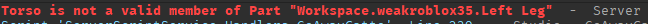# ACTUALLY getting character from .Touched

I’ve been trying to get humanoid from touched event when i clealry defined __HIT.Parent, which very much should clearly get the parent of humanoidrootpart, however it does not seem to work for whatever reason.

``````__WOODS.Touched:Connect(function(__HIT)
if __HIT:IsA("BasePart") and __HIT.Name ~= "Wood" and not __DEBO then
__DEBO = true
local __CHAR = __HIT.Parent
local __HUM = __CHAR:WaitForChild("Humanoid")
local BodyVelocity = Instance.new("BodyVelocity", __CHAR.Torso)
BodyVelocity.Velocity = Vector3.new(3,50,3) * 10
__HUM:TakeDamage(math.random(20,40))
__DEBO = false
end
end)
``````

My issue here is it gets Right Leg “humanoid” instead of the actual humanoid in the character model.

1 Like

You should not be waiting for the humanoid on touch, you should just be checking if the humanoid exists.

``````local character = hit.Parent
local humanoid = character:FindFirstChild("Humanoid")
if humanoid then
-- damage the humanoid, etc
end
``````
1 Like

Try this:

``````__WOODS.Touched:Connect(function(__HIT)
if __HIT.Name == "Wood" or __DEBO then
return
end

local __CHAR = __HIT.Parent
local __HUM = __CHAR:FindFirstChildWhichIsA("Humanoid")

if not __HUM then
return
end

__DEBO = true

local BodyVelocity = Instance.new("BodyVelocity")
BodyVelocity.Velocity = Vector3.new(3, 50, 3) * 10
BodyVelocity.Parent = __CHAR.Torso

BodyVelocity:Destroy()
end)

__HUM:TakeDamage(Random.new():NextInteger(20, 40))

__DEBO = false
end)
``````
1 Like
``````__WOODS.Touched:Connect(function(__HIT)
local __PLAYER = game:GetService("Players"):GetPlayerFromCharacter(__HIT.Parent)

if __PLAYER ~= nil and not __DEBO then
__DEBO = true
local __CHAR = __PLAYER.Character
local __HUM = __CHAR:WaitForChild("Humanoid")

local BodyVelocity = Instance.new("BodyVelocity")
BodyVelocity.Velocity = Vector3.new(3, 50, 3) * 10
BodyVelocity.Parent = __CHAR.Torso
__HUM:TakeDamage(math.random(20, 40))
__DEBO = false
end
end)
``````

see if this works

Tried this solution but i get this error nowLine thats causing it:

``````local BodyVelocity = Instance.new("BodyVelocity", __CHAR.Torso)
BodyVelocity.Velocity = Vector3.new(3,50,3) * 10
``````

Your current code is a terrible way to do it. Here’s some new code.

``````Woods.Touched:Connect(function(Hit)
local Player = game:GetService("Players"):GetPlayerFromCharacter(Hit.Parent)

if Hit.Parent:FindFirstChildOfClass("Humanoid") and Player and Hit.Parent:FindFirstChild("HumanoidRootPart") and not db then

db = true
local char = Hit.Parent
local hum = char:FindFirstChild("Humanoid")

local BodyVelocity = Instance.new("BodyVelocity", char.HumanoidRootPart)
BodyVelocity.Velocity = Vector3.new(3,50,3) * 10

hum:TakeDamage(math.random(20,40))
db = false
end
end)
``````

Also, I don’t understand why you put __ before everything… it makes it very hard to read…

Well the reason i do this it because stands out and makes it easy to get variables.

It overall decreases the readability of the code, and makes it hard to understand. At least for me.
Personally, I case my variables like so:

``````local runService = game:GetService("RunService") -- Services use camelCase
local PlayerAmount = 0 -- Variables use PascalCase
``````